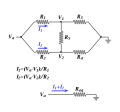# How do I calculate the equivalent resistence between terminals "A-B"

#### Diego Navarro

Joined Oct 24, 2016
1
Supose evrey resistor has a diffient value

#### WBahn

Joined Mar 31, 2012
25,545
That depends on what skills you have already learned.

Have you done anything with Wheatstone bridges or Delta-Wye transforms?

If you were to apply a constant voltage source across the terminals A and B, could you analyze the resulting circuit to determine how much current was flowing in the source?

•HunterDX77M

#### RBR1317

Joined Nov 13, 2010
543
In my opinion the reference ground is in the wrong place. While it should be possible to excite this resistor network with differential voltages, why complicate the analysis? That is assuming the solution is found via basic circuit analysis (start by finding V1 & V2) rather than the application of a delta-wye transform. Note that the answer I obtained is the sum of eight triple products divided by the sum of eight double products.#### HunterDX77M

Joined Sep 28, 2011
104
If this is for a first year circuits course then WBahn's suggestion is pretty much the textbook solution. Just apply a test source across A/B (like 1V to make the math easy) and then divide by the current flowing through terminals A/B to get the resistance. The current can be obtained using standard nodal/mesh analysis techniques that you've probably already learned.